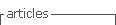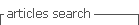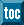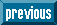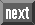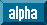## Indicators

•Cited by SciELO
•Access statistics

•Cited by Google
•Similars in SciELO
•Similars in Google

## Print version ISSN 0120-5609

### Ing. Investig. vol.34 no.1 Bogotá Jan./Apr. 2014

#### http://dx.doi.org/10.15446/ing.investig.v34n1.42797

http://dx.doi.org/10.15446/ing.investig.v34n1.42797

Procedure for calculating added value: application in the medium-voltage fuse industry

El procedimiento para calcular el valor agregado: una aplicación en la industria de fusibles de media tensión

A. Vinajera1, F. Marrero2 and W. A. Sarache3

1 Andrey Vinajera Zamora. Industrial Engineer, MSc and PhD (c). Affiliation: Asistant professor at Universidad Central "Marta Abreu" de Las Villas, Cuba.
Email: andreyvz@uclv.edu.cu

2 Fernando Marrero Delgado. Industrial Engineer, MSc and PhD. Affiliation: Head Professor at Universidad Central "Marta Abreu" de Las Villas, Cuba.
E-mail: fmarrero@uclv.edu.co

3 William Ariel Sarache. Industrial Engineer, MSc., PhD. Affiliation: Associate professor at Universidad Nacional de Colombia, Sede Manizales.
Email: wasarachec@unal.edu.co

How to cite: Vinajera, A., Marrero, F., Sarache, W. A., Procedure for calculating added value: application in the medium-voltage fuse industry., Ingeniería e Investigación, Vol. 34, No. 1, April, 2014, pp. 90 – 94.

ABSTRACT

This paper presents a procedure to calculate the added value of a process, through a pull manufacturing approach. The procedure allows calculating the selling price, the added value for each operation and the capital required to purchase the raw material. Additionally, the procedure sets the added value of the product for each operation, analyzing its increase or decrease based on the difference between the raw material cost and the purchasing budget. Finally, the results of its application in a medium-voltage fuse industry in the Cuban Republic are shown.

Keywords: Procedure, added value, push manufacturing system, processes.

RESUMEN

El presente artículo expone un procedimiento para calcular el valor agregado de un proceso, aplicando un enfoque de manufactura pull. Este permite calcular el precio para la venta, el valor agregado en cada una de las operaciones y la cantidad de dinero requerido para comprar la materia prima. Adicionalmente, el procedimiento permite establecer el valor agregado del producto en cada operación, analizando así, su aumento o disminución a partir de la diferencia entre el costo de la materia prima y el presupuesto de la compra. Finalmente, se muestran los resultados de su aplicación en una empresa industrial de fusibles de media tensión en la República de Cuba.

Palabras clave: procedimiento, valor agregado, sistema de manufactura push, procesos.

Received: June 11th 2013 Accepted: December 10th 2013

Introduction

Under current economic conditions, leading a company towards the customers' needs is necessary for survival in the business field. The customer service level depends on a successful integration of the company's processes, through the adoption of a logistic approach (Bayraktar et al., 2012). One of the most widely accepted manufacturing approaches has been the commonly called, pullsystem (Iwase and Ohno, 2011). In a production system, the pull concept implies that the production rate of a certain operation is controlled by the rate of the subsequent operation. This approach allows the company not only to improve its inventory level, but to also synchronize its system production rate according to the lower-capacity operation (bottleneck) (Ohno, 2011).

By applying a pull-system, it is possible to reduce costs and improve the service dimensions at the same time (Ghisi and Silva, 2006). However, organizing operations based on the desired customer service level is not an easy task; likewise, identifying operations that generate greater added value to the process, is an even more difficult task. Adding value is one of the most important aims of any production process. In economic terms, the added value is the economic value (additional value) acquired by products when they are transformed in the production process. Generally, the added value is associated with the concept of economic added value (Vogel, 2011).

There are various methodologies oriented to calculate the added value; however, according to the literature review performed by Sharma and Kumar (2010), most of them make global estimations based on the finished product value without taking into consideration the added value of each operation in the process; then, most of the existing contributions, do not identify the operations that generate greater added value, in order to prioritize improvement activities. For example, Xin'e et al. (2012) and Longinidis and Georgiadis (2013) performed estimations of added value in a global way; the first authors, in a banking organization and the latter, in an industrial supply chain. Meanwhile, Sathre and Gustavsson (2009) estimated the added value based on a detailed analysis of each operation, but without setting the input and output values for each of them.

Therefore, this paper shows a sixstage procedure for added value calculation under a pull manufacturing approach. The procedure allows us to calculate the added value for each operation of the process. Likewise, it allows identification of the most important operations (bottleneck), where improvement actions should be emphasized in order to increase the process performance. In this way, the procedure is consistent with the contributions of Goldratt and Fox (1994) and Goldratt and Cox (1995), regarding the postulates of the theory of constraints (TOC).

The procedure was applied in a production process of a medium-voltage fuse company, located in Santa Clara (Cuba). The results allowed for the establishment of the hierarchical importance of the operations, calculation of the added value in each of them, evaluation of the possibility of combining some operations and estimation of the monetary resources needed for raw material purchasing.

The added value is defined as the difference between the out-put value and input value of a product after going through an operation or process (Ketchen Jr and Hult, 2007; Mikkonen, 2011). This concept is close to the economic added value concept (EVA) proposed by Baudin (2011), which calculates the difference between net operating profit after interest and company investment. However, under a pull manufacturing approach, the value per each operation must be quantified in order to identify the key activities and operations in order to reduce costs.

Therefore, the economic added value to an operation according to its relative importance within the process, is the concept that best fits the approach of the present article. This concept is strongly linked to the value chain concept. The value chain is defined as an integration process of operations and resources of the manufacturing system in order to add value to a product or service (Ketchen Jr and Hult, 2007; Torres-Fuchslocher and de-la-Fuente-Mella, 2009; Walters, 2012).

Under this concept, it is important to calculate the added value for each operation so as to identify critical points and establish cost reduction alternatives. Fig. 1 presents a conceptual model of the situation. In it, Xik refers to the added value for product k in operation i (i = 1, n) and VFk and PVk refer to the final value and selling price of product k, respectively.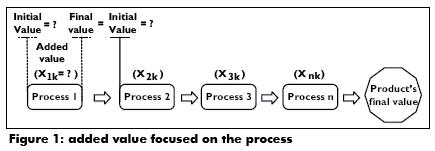On the other hand, the economic value is created only when the customers are willing to pay a price that overcomes the produc-tion cost (Baudin, 2011), it is possible to establish the added value, onxly when the product is finished (Zirkler, 2002). To address this issue, DaSilva (2005) proposed two types of added value: the added value to the product and the net added value. The first is defined by the added cost to the product (components, modifications, etc.) and the latter refers to the obtained profit.

Fig. 2 shows the situation described above; in it, Xik refers to the added value to product k in operation i. Respectively, VIik and VFik represent the initial and final values of product k in operation i. In turn, the final value of an operation is equal to initial value of the following (VI1+ik); CAik is the added cost to product k in the operation i; XFk is the total added value to product k; Pvk is the selling price of product k; Xnk is the net added value to product k and finally, Wik represents the weight or relative importance of operation i in product k. The operation weight affects the net added value and it depends on the costs, processing times and inventory level.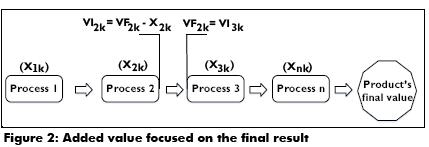Proposed procedure

The proposed procedure is based on a pull manufacturing approach and it consists of six steps described as follows.

Step 1. Definition of the product's selling price. The product's selling price is a decision that depends on both production costs and competitors prices (Fletcher and Russell-Jones, 2002). Cost is a function of used resources to get the product (Nagle and Holden, 1995). Meanwhile, competitors prices become a point of reference for pricing not only based on cost, but also in added value (Fletcher and Russell-Jones, 2002). Then, the issue is to define a appropriate selling price able to cover production costs and at the same time, be competitive in the market.

Step 2. Identification of process operations. A process is a coordinated system of operations through which the product obtains an added value (Walters, 2012). Each operation must be identified according to its production capacity, technology, layout and working methods. In the current procedure, the operation capacity can be calculated using Equation 1.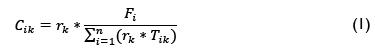Where:

Cik: capacity of operation i in product k

rk: specific weight of product k in the total production volume

Tik: Time of operation i in product k

Fi: available time for operation i

n: number of products to be manufactured in operation i

Step 3. Identification of operational requirements. According to Marrero-Delgado (2001), at this stage the limit conditions and requirements of each operation must be established, taking into account the list of materials, components and other required resources for the process.

Step 4. Calculation of the added value coefficient. At this step, the added value in each operation is calculated. The added value to operation i (Xi) depends on the cost and operation time. The added value coefficient represents a product cost percentage at each stage of the process. The calculation of this coefficient can be performed by using the following procedure:

a) Characterization of process operations. Operation characteristics, machinery and the operations components must be identified. Operations can be mechanical, mechanical-manual or manual.

b) Calculation of operation time. Depending on the operation type, the operation time can be calculated by using Equations 2, 3 or 4 as follows: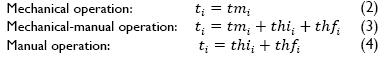where ti is the product time in operation i; tmi is the machining time in operation i; thi is the component preparation time; thfi is the time of final activities when the product leaves the machine.

c) Calculation of added value. As is shown in Equation 5, the added value to an operation consists on the added value by the machine or equipment (Xmik), the materials or components (Xcik) and the operators (Xhik).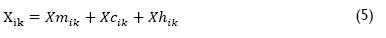The added value to the process is calculated by using equations 6 to 8, where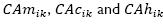represent the added cost by machines, components and operators respectively; in turn,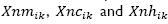represent the net added value by machines, components and operators respectively.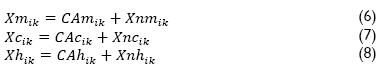The added value to product k in operation i (Xik), is calculated by using Equation 9, where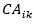represents the added costs to product k in operation i and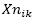is the net added value to product k in operation i. In turn, by applying Equation 10, it is possible to calculate the net added value to product k in operation i (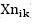), where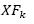represents the final value of product k (selling price) and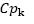is the production cost of product k, which can be obtained by applying Equation 11 (Cmpk is the initial raw material cost and n is the total number of operations in the process).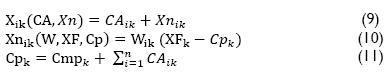The relative importance of operation i in product k (Wik) can be calculated through Equation 12, where CDik and CIik are, respectively, the direct and indirect cost of product k in operation i; CDTk and CITk represent the direct and indirect cost of the entire process; tik is the processing time of product k in operation i; Tik is the total processing time of product k; Invik is the inventory level in operation i and Invk is the total work in process of product k. Although in a pull manufacturing system, the production rate of an operation depends on the rate of the next one, sometimes a minimum inventory level to hold the material flow is required.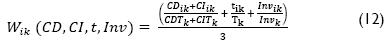The ratio between the obtained profits and selling price (WGk) can be calculated by using Equation 13, where Cpk is the production cost of product k (Equation 11); Pvk is the selling price of product k and Cnp represents the nonassociated cost to the process.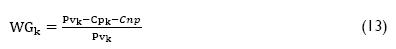Moreover, because in bottleneck operations the greatest inventory level takes place, both variables tik and Invik in Equation 12, are directly proportional to the relative weight. Therefore, the operation of the greatest net added value is the bottleneck, which may or may not coincide with the operation of higher added value. This situation occurs because it is not always the the case that the highest percentage of an operation cost belongs to the aggregate costs, which directly influences its added value.

Step 5. Calculation of value for each product at each operation. The value of each product at each operation is calculated by applying Equation 14, where VIik is the initial value of product k in operation i. Also, the final value of product k in any operation is equal to the initial value in the following operation (VFik=VI1+ik).

>

Step 6. Calculation of the required capital for purchase of raw material. The amount of money required to buy the raw material is equal to the initial value of the first operation of the process (VI1). The raw material cost must be less than VI1; otherwise, if the company decides to buy it in order to meet the delivery time, the coefficients of the added value must be modified. In this case, Cmpnk becomes the new raw material initial cost (VIn1). While the difference between Cmpk and VI1 persists, the value of Xnik will increase or decrease. Moreover, the coefficients of added value per each process must be recalculated by using Equation 9, where CAik would remain constant and Xnik would change, affecting each of the aggregated values previously calculated. At the same time, it must be taken into consideration that the difference between the purchasing cost of raw materials and VI1 should be less than the product profit.

On the other hand, regarding the selling price, two possible scenarios could occur; the first corresponds to a fixed price and the second to a variable price. In the first scenario, XFk would not be affected because the difference would only affect the product´s net profit and, therefore, the values of Xnik and Xik would be affected as well. Subsequently, the new net added value (XNnik) and each operation added value must be recalculated by applying Equations 9 or 10; in this case, Xnik must be replaced by XNnik which could be calculated by using Equations 15 and 16 as follows: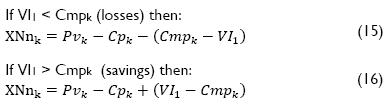In the second scenario, due to variations in price, if the company wants to constantly obtain the same profit (Pvk-Cmpk), the new added value for each operation should be calculated by applying Equation (9) and the new XFnk by using Equations 17 or 18 as follows: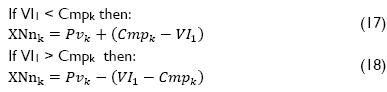Results

The procedure was applied at an electromechanical products company located in Santa Clara (Cuba), specifically in the medium-voltage fuse process, with the following results:

Step 1. Definition of the product's selling price. In the selected process, two types of fuses are manufactured, which differ from each other depending on the voltage (15 KV and 34 KV). In this paper, the 15 KV and 1 ampere fuse were selected as examples to apply the proposed procedure. The product's selling price is \$1.93 according to the rates established by the government.

Step 2. Identification of process operations. In the selected process, some operations are semiautomatic and others are manual. The list of operations and their processing times are shown in Table 1. The capacity was determined by Equation 1 (Fi = 1 hour). The specific weight of each product (rk) was 2.5%. As seen in Table 1, operation 7 (assembly) is the bottleneck of the process.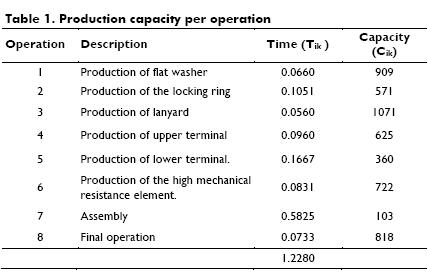Step 3. Identification of operational requirements. Table 2 shows the list of materials, components and other required resources for the process.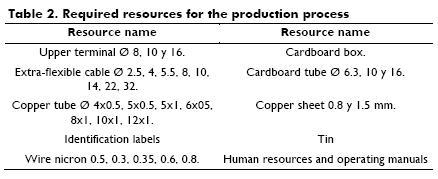Step 4. Calculation of the added value coefficient. The cost and inventory level for each operation are shown in Table 3. The operation times (Tik) are the same shown in Table 1. According to a previous analysis, operation 8 was classified as a manual operation and the remaining as mechanical-manual operations. The data used to calculate the added values at each operation are also shown in Table 3. As seen in the table, the added costs of the process correspond to a value of \$ 0.458, profits are \$ 0.160, in-direct costs are \$ 1.2874 and the remaining represents the raw material cost. Also, the bottleneck is the operation with the high-est net added value. Finally, by applying equation 13, the ratio be-tween the obtained profits and selling price (WGk) was 20%.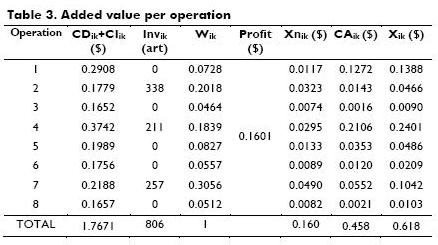Step 5. Calculation of value for each product at each operation. Table 4 shows the obtained added value at each operation. As shown, the final value of the product at any operation is equal to the initial value in the following operation. For example, 1.4504 is the final value at operation 1 and the initial value at operation 2.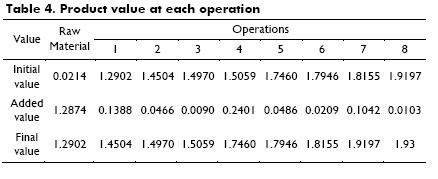Step 6. Calculation of the required capital for purchase of raw materials. In the case under analysis, a fixed cost scenario was assumed. Subsequently, indirect costs were subtracted from the initial value of the first operation (\$1.2902-\$1.2874). This out-come represents the required amount of money to purchase the raw material (\$ 0.0214). As this result was coincident with the raw material cost, it was not necessary to recalculate a new added value. However, for illustrative purposes, in Tables 5 and 6 the results of a sensitivity analysis to evaluate the effect on added value are shown. Table 5 shows the results of increasing one cent in the initial cost; in turn, Table 6 shows the results of decreasing the same amount.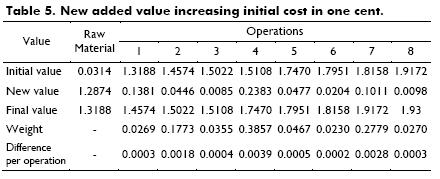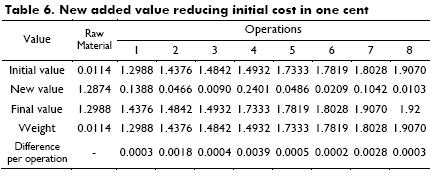Indirect costs (\$ 1.2874) are not part of any added cost (CAik); therefore, these costs are subtracted from the initial value of the first operation, resulting in the available money to buy the raw material. According to the data of Table 5, if the raw material cost is increased, the added value in each operation is decreased. This result is obtained by multiplying the operation´s weight and that increase; for example in the operation 1, the amount to be decreased is obtained by multiplying 0.0728 * \$ 0.01. Otherwise, if the raw material cost decreases, the added value is increased by applying the same procedure. In Tables 5 and 6 one can see that the difference per operation is the same, since the variation (increase or decrease) is \$ 0.01.

The same analysis should be done regardless the increase or decrease of the initial cost, subject to this amount does not exceed the product profit in case of increase (from 0 to 0.1602 cents) or in case of decrease (from 0 and 0.0214 cents). The increase or decrease of the initial cost inversely affects the profit and, in the same way, it affects the net added value of each operation. From another point of view, it is possible to analyze the distribution of this difference based on the relative importance (weight) of each operation. All of the above is valid in case of fixed price sales, because for sale prices and variable costs, the added value is affected in a direct way.

Conclusions

The proposed procedure is shown as a useful tool for both the calculation of added value and the identification of critical operations in a manufacturing process. From setting the selling price and through a pull approach, the procedure allows to calculate the added value in a production process, independently of the initial raw material cost and product profit. The procedure can be applied to any production system, since the added value calculation is done for each product regardless the operations sequence. Moreover, the calculated initial value allows comparisons with the purchasing budget, providing additional decision criteria (purchasing capacity) to the supplier's selection.

In the case of an operation or a machine dedicated to various products, the equipment operation cost will be the same for all production runs, varying only in processing times, inventory levels and the costs of the components. This is enough to get a different weight and added value for each operation and product.

In the selected process, it was demonstrated that the bottleneck (operation 7, assembly) was the operation of higher net added value. However, the operation of higher added value was the production of upper terminal (operation 4). Hence it is possible to state that the added value mainly depends on the operation´s relative weight and the net profit; in turn, the operation´s relative weight depends on the processing time, inventory level and related costs.

According to the results, the weight of the bottleneck operation was 30.56%. However, it must be established that after some syn-chronization efforts, the bottleneck operation could be changed. In this case, the aggregated values must be recalculated, because a new bottleneck requires adjustments in processing times, inventory levels and costs.

The analysis of the new aggregated values must be addressed in two ways. First, it is necessary to establish if the change of the added value is reflected in savings or economic loss; then, the added value for each operation should be increased in case of savings or reduced in case of loss, proceeding in the same manner as the net income was distributed.

Finally, when simultaneous changes happen (increase or decrease) in both the initial cost and the selling price, the effect of such change on operations must be identified. If the change´s effects are equal (savings or loss), they must be added; otherwise must be subtracted. After obtaining a single value, the obtained result should be distributed in each operation.

References

Baudin, C., Criterios de transferibilidad del enfoque concurrente en los procesos de diseño y desarrollo de productos de las pequeñas y medianas empresas chilenas., Ingeniare. Revista chilena de ingeniería, Vol. 19, No.1, 2011, pp. 146-161.         [ Links ]

Bayraktar, E., Tatoglu, E., Turkyilmaz, A., Delen, D., Zaim, S., Measuring the efficiency of customer satisfaction and loyalty for mobile phone brands with DEA., Expert Systems with Applications, Vol. 39, No.1, 2012, pp. 99-106.         [ Links ]

DaSilva, C. L., Competitividade internacional da indústria brasileira exportadora de papel de imprimir e escrever à luz da cadeia de valor., Revista do Producao, Vol. 15, No.1, 2005, pp. 114-126.         [ Links ]

Fletcher, T., Russell-Jones, N., Política de precios: cómo crear el máximo valor a través de una fijación de precios efectiva., España, Deusto, 2002.         [ Links ]

Ghisi, F. A., Silva, A. L. D., Implantação do Efficient Consumer Response (ECR): um estudo multicaso com indústrias, atacadistas e varejistas., Revista de Administração Contemporânea, Vol. 10, No.3, 2006, pp. 111-132.         [ Links ]

Goldratt, E. M., Fox, J., La carrera., Mexico D.F., Ediciones Castillo, 1994, pp. 1-250.         [ Links ]

Goldratt, E. M., Cox, J., La meta. Un proceso de mejora continua., Mexico D. F., Ediciones Castillo,1995, pp. 1-407.         [ Links ]

Iwase, M., Ohno, K., The performance evaluation of a multistage JIT production system with stochastic demand and production capacities., European Journal of Operational Research, Vol. 214, No. 2, 2011, pp. 216-222.         [ Links ]

Ketchen Jr, D. J., Hult, G. T. M., Bridging organization theory and supply chain management: The case of best value supply chains., Journal of Operations Management, Vol. 25, No. 2, 2007, pp. 573-580.         [ Links ]

Longinidis, P., Georgiadis, M. C., Managing the tradeoffs between financial performance and credit solvency in the optimal design of supply chain networks under economic uncertainty., Computers & Chemical Engineering, Vol. 48, No. 0, 2013, pp. 264-279.         [ Links ]

Marrero-Delgado, F., Procedimientos para la toma de decisiones logísticas con enfoque multicriterio en la cadena de corte, alza y transporte de la caña de azúcar. Aplicaciones en el CAI de la provincia de Villa Clara., A doctral Thesis presented at Universidad Central Marta Abreu de Las Villas, Santa Clara, Cuba, 2001.         [ Links ]

Mikkonen, K., Exploring the creation of systemic value for the customer in Advanced Multi-Play., Telecommunications Policy, Vol. 35, No. 2, 2011, pp. 185-201.         [ Links ]

Nagle, T. T., Holden, R. K., Estrategia y tácticas para la fijación de precios: Guía para tomar decisiones beneficiosas., Buenos Aires, Granica S. A., 1995, pp. 42-73, 122-187.         [ Links ]

Ohno, K., The optimal control of justin-time-based production and distribution systems and performance comparisons with optimized pull systems., European Journal of Operational Research, Vol. 213, No.1, 2011, pp. 124-133.         [ Links ]

Sathre, R., Gustavsson, L., Process-based analysis of added value in forest product industries., Forest Policy and Economics, Vol. 11, No.1, 2009, pp. 65-75.         [ Links ]

Sharma, A. K., Kumar, S., Economic value added (EVA)-literature review and relevant issues., International Journal of Economics and Finance, Vol. 2, No. 2, 2010, pp. 200-215.         [ Links ]

Torres-Fuchslocher, C., de-la-Fuente-Mella, H., Factores de éxito en proveedores de bienes manufacturados de la salmonicultura chilena., Ingeniare. Revista chilena de ingeniería, Vol. 17, No.1, 2009, pp. 58-67.         [ Links ]

Vogel, H.-A., Do privatized airports add financial value?, Research in Transportation Business & Management, Vol. 1, No.1, 2011, pp. 15-24.         [ Links ]

Walters, D., Competition, Collaboration, and creating value in the value chain, modelling value., Springer, 2012, pp. 3-36.         [ Links ]

Xin'e, Z., Ting, W., Yuan, Z., Economic value added for perfor-mance evaluation: A financial engineering., Systems Engineering Procedia, Vol. 5, 2012, pp. 379-387.         [ Links ]

Zirkler, B., Der Economic Value Added (EVAR®) als Konzept für den Mittelstand., Controlling & Management, Vol. 46, No. 0, 2002, pp. 98-104.         [ Links ]